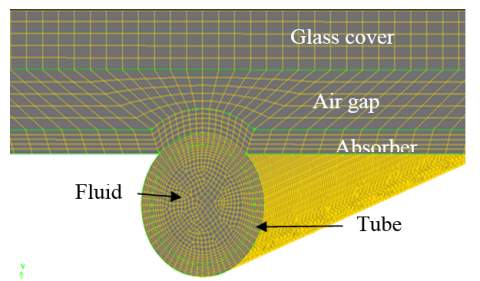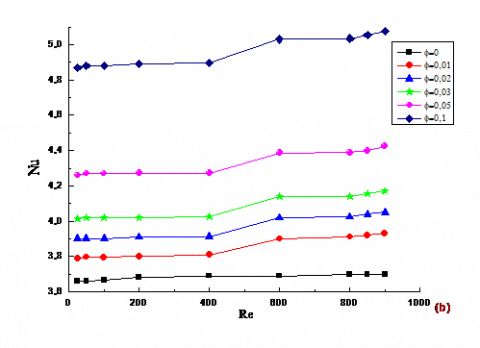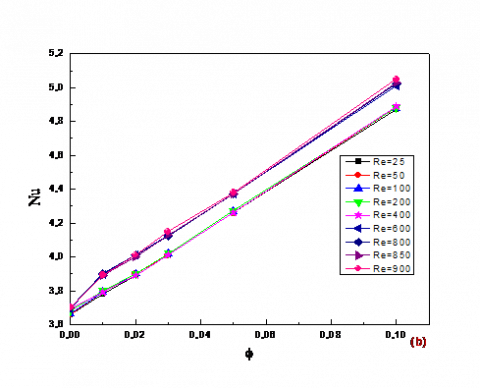# Numerical Analysis on Energy Transfer in A Flat Plate Collector Using SiO2

Numerical Analysis on Energy Transfer in A Flat Plate Collector Using SiO2

Department of Chemical Engineering, Boston College, United State of America

Corresponding Author Email:
okulov.valerry1972@gmail.com
Page:
6-11
|
DOI:
https://doi.org/10.18280/psees.030102
Received:
9 September 2019
|
Accepted:
25 December 2019
|
Published:
30 December 2019
| Citation

OPEN ACCESS

Abstract:

This paper illustrates how practical application of nanoparticles (SiO2) as working fluid to stimulate solar flat plate collector efficiency with heat transfer modification properties. A numerical study of nanofluids laminar forced convection, permanent and stationary (SiO2), is conducted in a solar flat plate collectors. The effectiveness of these nanofluids are compared to conventional working fluid (water), where in the dynamic and thermal properties are evaluated for four volume concentrations of nanoparticles (1%, 3%, 5% and 10%), and this done for   Reynolds number from 25 to 800. Results from the application of those nonfluids are obtained versus average temperature; pressure drop coefficient and Nusselt number are discussed later in this paper. Finally we concluded that heat transfer increases with increasing both nanoparticles concentration and Reynolds number.

Keywords:

forced convection, CFD, nanofluid, heat transfer, SiO2 nanoparticles, solar flat plate collectors

1. Introduction

Thermal solar energy has a major importance in the world because it’s clean, renewable and sustainable, it has several practical and industrial applications, including electrical production, energy systems heating and cooling; in which the principal elements in these systems are the solar collectors which convert  solar radiation to  thermal energy then transfer it towards the carrying fluid. The most known solar collectors used are the flat-plat collector. However, this type has relatively low energy efficiency and low outlet temperatures. Recently, several studies have been carried out on the flat plate solar collector to overcome these disadvantages [1-3], in addition to these classical studies or methods, which are used to increase the thermal efficiency of this kind of solar collector, one of the most latest effective method is to replace the conventional working fluid by other fluids, which having higher thermal properties.

The idea to improve thermo-physical properties of conventional fluids is the adding solid particles with a high thermal characteristics and nano-size, inside basic fluid. This new generation of fluids named Nanofluids; this term was introduced first by Choi , and usually stills use to characterize this colloidal suspension type. Subsequently, numerous experimental and numerical studies have been carried out by several researchers [5-7], to understand and analyze thermo-hydraulic behavior of nanofluids. First, an experimental studies are done on the thermo-physical properties of nanoluides Eastman , Choi et al  and Patel et al , which observe, that the thermal conductivity of nanofluids are very high compared to that of  conventional fluid and exceed those predicted by conventional approaches. After these results, Keblinski et al , Jang and Choi  and then Evans  developed thermal conductivity classic model of the nanofluid, where they introduced the effect of the Brownian motion and nanoparticles agglomeration effect, which explain the important improvement.

Sankar  studied the effect of heat convection between the nanoparticles induced by the Brownian movement, and noted that the model of Koo and Kleinstreuer  ,Jang and Choi  is more valid than this of Evans and Eapen . Despite these studies which have been realized to improve heat transfer by using the nanofluids in the last decade, till know, does not exist a commercial solar collector using these fluids. However, an experimental study on the solar water heater Natarajan et al. , found that if the nanofluids are used as working fluids, the energy efficiency of solar water heaters increases significantly compared to conventional fluids.

On the other hand, the dispersion of the nanoparticles gives an important increase in the heat transfer coefficient [15-20]. Hence, they found that it is possible to reduce the exchange surface and improve the efficiency of these devices.

In this paper, we present the study of heat transfer of nanofluid (SiO2) flows through a solar flat plate collector. In the first part, we describe the problem and present the boundary conditions. After grid independence study, then we validated the results by comparing them with previous literature reports.

The key part of this work is to involve the simulation with the interpretation of numerical results obtained for this case, where the effect of nanoparticles are shown for various volume concentration and Renolds number, and studied systematically. At the end, this paper is footed by conclusion which summarizes the main results obtained.

2. Mathematical Formulation

The flow domain consists of an absorber plate and circular absorber tube.  The absorber plate is covered with glass plate with an air gap. Design parameters and fixed geometric parameters have been taken similar to Karanth et al. , as indicated in Table. I.

The forced heat transfer of nanofluide under laminar flow inside the absorbent tube, as shown in Fig. 1, the flow is assumed steady and the ﬂuid possesses uniform axial velocity V0 and temperature T0 proﬁles at the inlet of tube.

2.1 Assumptions

The nanoparticles used in practical applications of heat exchange are very thins (≤ 50 nm), then the liquid-particle mixture may easily be fluidized and considered as a homogenous single-phase fluid .

By assuming negligible thermal equilibrium and slip between the phases, the apparent thermal properties of nanofluids can be estimated by using classical relationships of two-component mixture , and the mixture considered as a conventional single-phase ﬂuid .

Table 1. Design parameters of solar collector

 absorber plate (mm) Tube (mm) glass plate (mm) air gap (mm) length 1 000 1010 1000 1000 wide 150 - 150 150 diameter - 10 - thickness 2 1 2 3

2.2 Governing equations and boundary condition

Under the previous assumptions, the general governing equations are:

2.2.1 Continuity equation

$\frac{\partial}{\partial x_{j}}\left(\rho_{n f} u_{j}\right)=0$   (1)

2.2.2 Momentum equation

$\rho_{n f} \frac{\partial\left(u_{i} u_{j}\right)}{\partial x_{j}}=-\frac{\partial P}{\partial x_{i}}+\frac{\partial}{\partial x_{j}}\left(\mu_{n f} \frac{\partial u_{i}}{\partial x_{j}}\right)$    (2)

2.2.3 Energy equation

$\frac{\partial}{\partial x_{j}}\left(u_{j} T\right)=\frac{k_{n f}}{\rho_{n f} C p_{n f}}\left(\frac{\partial^{2} T}{\partial x_{j}^{2}}\right)$    (3)

The physical propertiesof nanofluid, nanofluid densityρnf , viscosity μnf , Thermal conductivity knf  and Nanofluid specific heat Cpnf  are given with the below equations:

$\rho_{n f}=\rho_{s} \phi+\rho_{f}(1-\phi)$    (4)

$\mu_{n f}=(1+2.5 \phi) \mu_{f}$    (5)

$k_{n f}=\frac{k_{s}-2 k_{f}-2 \phi\left(k_{f}-k_{s}\right)}{k_{s}+2 k_{f}+\phi\left(k_{f}-k_{s}\right)} k_{f}$    (6)

$C p_{n f}=\frac{\rho_{s} C p_{s} \phi+\rho_{f} C p_{f}(1-\phi)}{\rho_{n f}}$    (7)

For boundary conditions and operating parameters are taken similar to Karanth et al. . For inlet a ‘velocity inlet’ boundary condition is specified and an ‘outflow’ condition is specified at the outlet for the water domain. The glass material is made up of Borosilicate, which has a refractive index of 1.47. Absorber plate and absorber tube are made up of copper material. Wall boundary conditions used to bound fluid and solid regions. The interface between water and absorber tube is defined as coupled wall condition. In the top of collector a constant solar heat flux equal to 800W/m2 are imposed.

Table 2. Properties of various used material

 Properties Absorber P&T Glass Plate Air ρ (kg/m3) 8978 2230 1.225 Cp (J/kg°k) 381 750 1006.4 K (W/m°k) 387.6 1.14 0.0242
3. Mathematical Formulation

To conduct numerical simulation, the computational domain was meshed with control volumes built around each grid using GAMBIT (version 2.4.6), which is the pre-processor for FLUENT (version 6.3). Numerical simulation was carried out using steady state implicit pressure based solver, which is an in-built in the commercially available software FLUENT (version 6.3). Governing partial differential equations, for mass and momentum, are solved for the steady incompressible flow. The velocity-pressure coupling is affected through SIMPLE algorithm developed by Patankar . Second order upwind schemes were chosen for the solution schemes laminar flow condition was used.

Computational domain was meshed and done by using appropriate grid cells, with suitable size. Three dimensional computational domains are built and used, as shown in Figure 1.

To solve the previous system of governing equations (1-3), the computational domain was meshed with the ﬁnite control volume method  witch has been successfully used by several authors [29-30]. To compute the combined convection-and-diffusion ﬂuxes of heat the upwind method, where the upwind scheme of the second order has been used throughout for computing heat and momentum fluxes.

## 1.pngFigure 1. Mesh of the computational domain

Several non-uniform grids have been thoroughly tested to ensure the accuracy and the consistency of numerical results, which has shown that the 40 x 60x 500 non-uniform grid (32, 24 and 155 nodes, respectively, along the radial, tangential and axial directions) appears satisfactory for the absorber tube; with refining mesh near the tube wall and the entrance region.

The numerical results of the contour temperature distribution are presented in figure 2, and the comparison of fluid temperature at axial distance for various axial velocities between this study and these of Karanth et al  are illustrated in figure 3.

The numerical results obtained by Karanth et al, which provide an excellent test tool to validate our calculation code; by comparing these two curves, we can see good match between our results and those of the authors.

4. Results and Discussion

We study the nanofluids heat transfer flow inside an absorbent tube, where he heated by the absorbent plaque. We consider the flow regime as laminar, and varying the number of Reynolds between 25 and 900, for the different nanoparticles concentrations (1%, 3%, 5%, and 10%). Than we plot the results, pressure drop coefficient and Nusselt number.

## 2a.png## 2b.png## 2c.png## 2d.pngFigure 2. Temperature distribution on the absorber: (a) V= 0.001 m/s, (b) V= 0.005 m/s, (b) V= 0.01 m/s, (b) V= 0.05 m/s

## 77.pngFigure 3. Comparison of fluid temperature distribution between this study and these of Karanth et al 

4.1 Pressure drop coefficient

Figures 4-(a) and 4-(b), represent the variation of pressure drop coefficient versus volume concentration "$\phi$" and Reynolds number "Re". We note that the coefficient λ remains practically constant for all nanoparticles concentrations.

This result, therefore, reflects the insignificant influence of the nanoparticles volume concentration on the pressure drop coefficient λ. This result was predictable.

## 4.png(a)                                                   (b)

Figure 4. Pressure drop coefficient versus: (a) volume fraction of SiO2nanoparticles and (b) Reynolds numbers

4.2 Nusselt number

Figures 5-(a) and 5-(b), represents the Nusselt number "Nu" evolution as a function of Reynolds number and the volume fraction "$\phi$".

We can note on the one hand, a significant increase in the number of Nusselt with the increase in nanoparticle volume concentration, so in relation with pure water, and on the other hand, a slight increase versus Reynolds number.

In the Figure 6, represent the comparison in term of rate improvement of heat transfer coefficient for various volume concentrations of nanoparticles.We notice then, a considerable increase of the improvement rate compared topure water which can reach anaverage value of 34 % for $\phi$= 10 %.

## 5a.png(a)

## 5b.png(b)

Figure 5. Heat transfer coefficient versus: (a) Reynolds number and (b) different concentrations of SiO2nanoparticles

## 6.pngFigure 6. The improvement rate of heat transfer coefficient (%)

5. Conclusion

In this paper, the flow and heat transfer characteristics of SiO2-water nanofluid for arrange of the Reynolds number (25to 900) with a wide range of volume concentration (0 to 10%) are studied numerically.

We can confirm from analyze of thermal-hydraulic properties of nanofluids, on one hand, the presence of nanoparticles in the base fluid (pure water) increases significantly Nusselt number, that is also growing with the increase of volume fraction. This increase in heat coefficient (Nu) results in a good heat transfer between the energy systems compared to the base fluid (pure water) case. On the other hand, the gain in heat (increase in Nu) is unfortunately accompanied by a slight increase in pressure drop coefficient (λ).

The thermo-physical properties of nanofluids are considered constant. The results show that the both, Nusselt number and heat transfer coefficient, of nanofluid are strongly dependent on nanoparticle and increase by increasing of the volume concentration of nanoparticles. Also the results illustrate that by increasing the volume concentration, the shear stress increase.

References

 Martín, R.H., Pérez-García, J., García, A., García-Soto, F.J., López-Galiana, E. (2011). Simulation of an enhanced flat-plate solar liquid collector with wire-coil insert devices. Solar Energy, 85(3): 455-469. https://doi.org/10.1016/j.solener.2010.12.013

 García, A., Martin, R.H., Pérez-García, J. (2013). Experimental study of heat transfer enhancement in a flat-plate solar water collector with wire-coil inserts. Applied Thermal Engineering, 61(2): 461-468. https://doi.org/10.1016/j.applthermaleng.2013.07.048

 Sandhu, G., Siddiqui, K., Garcia, A. (2014). Experimental study on the combined effects of inclination angle and insert devices on the performance of a flat-plate solar collector. International Journal of Heat and Mass Transfer, 71: 251-263. https://doi.org/10.1016/j.ijheatmasstransfer.2013.12.004

 Choi, S.U.S., Eastman, J.A. (1995). Enhancing thermal conductivity of fluids with nanoparticles (No. ANL/MSD/CP-84938; CONF-951135-29). Argonne National Lab., IL (United States).

 Eastman, J.A., Choi, S.U.S., Li, S., Yu, W., Thompson, L.J. (2001). Anomalously increased effective thermal conductivities of ethylene glycol-based nanoluids containing copper nanoparticles. Applied physics letters, 78(6): 718-720. https://doi.org/10.1063/1.1341218

 Choi, S.U.S., Zhang, Z.G., Lockwood, F.E., Yu, W., Grulke, F.A. (2001). Anomalous thermal conductivity enhancement in nanotube suspensions. Applied physics letters, 79(14): 2252-2254. https://doi.org/10.1063/1.1408272

 Patel, H.E., Das, S.K., Sundararajan, T., Sreekumaran Nair, A., George, B., Pradeep, T. (2003). Thermal conductivities of naked and monolayer protected metal nanoparticle based nanoluids: manifestation of anomalous enhancement and chemical effects. Appl Phys Lett, 83(14): 2931-2933. https://doi.org/10.1063/1.1602578

 Keblinski, P.. Phillpot, S., Choi, S.U.S., Eastman, J.A. (2002). Mechanisms of heat low in suspensions of nano-sized particles (nanoluids). International journal of heat and mass transfer, 45(4): 855-863. https://doi.org/10.1016/S0017-9310(01)00175-2

 Jang, S.P., Choi, S.U.S. (2004). Role of Brownian motion in the enhanced thermal conductivity of nanoluids. Applied physics letters, 84(21): 4316-4318. https://doi.org/10.1063/1.1756684

 Evans, W., Fish, J., Keblinski, P. (2006). Role of brownian motion hydrodynamics on nanofluid thermal conductivity. Applied physics letters, 88(9): 093116. https://doi.org/10.1063/1.2179118

 Sankar, N., Mathew, N., Sobhan, C. (2008). Molecular dynamics modeling of thermal conductivity enhancement in metal nanoparticle suspensions. International Communications in Heat and Mass Transfer, 35(7): 867-872. https://doi.org/10.1016/j.icheatmasstransfer.2008.03.006

 Koo, J., Kleinstreuer, C. (2004). A new thermal conductivity model for nanoﬂuids. SpringerJournal of Nanoparticle Research, 6: 577-588. https://doi.org/10.1007/s11051-004-3170-5

 Eapen, J., Rusconi, R., Piazza, R., Yip, S. (2010). The classical nature of thermal conduction in nanoluids. J. Heat Transf, 132(10): 1024021-14. https://doi.org/10.1115/1.4001304,

 Natarajan, E., Sathish, R. (2009). Role of nanofluids in solar water heater. International Journal of Advanced Manufacturing Technology, 45: 1-5. https://doi.org/10.1007/s00170-008-1876-8

 Sokhansefat, T., Kasaeian, A.B., Kowsary, F. (2014). Heat transfer enhancement in parabolic trough collector tube using Al2O3/synthetic oil nanofluid. Renewable and Sustainable Energy Reviews, 33: 636-644. https://doi.org/10.1016/j.rser.2014.02.028

 Otanicar, T.P., Phelan, P.E., Prasher, R.S., Rosengarten, G., Taylor, R.A. (2010). Nanofluid-based direct absorption solar collector. Journal of  Renewable and Sustainable Energy, 2(3): 033102. https://doi.org/10.1063/1.3429737

 Faizal, M., Saidur, R., Mekhilef, S., Alim, M.A. (2013). Energy, economic and environmental analysis of metal oxides nanofluid for flat-plate solar collector, Energy Conversion and Management, 76: 162-168. https://doi.org/10.1016/j.enconman.2013.07.038

 Said, Z., Sajid, M.H., Alim, M.A., Saidur, R., Rahim, N.A. (2013). Experimental investigation of the thermophysical properties of AL2O3-nanofluid and its effect on a flat plate solar collector. International communications in heat and mass transfer, 48: 99-107. https://doi.org/10.1016/j.icheatmasstransfer.2013.09.005

 He, Q., Zeng, S., Wang, S. (2015). Experimental investigation on the efficiency of flat-plate solar collectors with nanofluids. Applied Thermal Engineering, 88: 165-171. https://doi.org/10.1016/j.applthermaleng.2014.09.053

 Menbari, A., Alemrajabi, A.A., Rezaei, A. (2016). Heat transfer analysis and the effect of CuO/Water nanofluid on direct absorption concentrating solar collector. Applied Thermal Engineering, 104: 176-183. https://doi.org/10.1016/j.applthermaleng.2016.05.064

 Karanth, K., Manjunath, M., Sharma, N. (2011). Numerical simulation of a solar ﬂat plate collector using discrete transfer radiation model (DTRM)-a CFD approach. In: Proceedings of the world congress on engineering, London, 3: 2355-2360.

 Xuan, Y., Roetzel, W. (2000). Conception for Heat Transfer Correlation of Nanofluids. International Journal of heat and Mass transfer, 43(19): 3701-3707. https://doi.org/10.1016/S0017-9310(99)00369-5

 Pak, B.C., Cho, Y.I. (1998). Hydrodynamic and heat transfer study of dispersed fluids with submicron metallic oxide particles. Experimental Heat Transfer, 11(2): 151-170. https://doi.org/10.1080/08916159808946559

 Zhang, X., Gu, H., Fujii, M. (2007). Effective thermal conductivity and thermal diffusivity of nanoﬂuids containing spherical and cylindrical nanoparticles. Experimental Thermal and Fluid Science, 31(6): 593-599. https://doi.org/10.1016/j.expthermflusci.2006.06.009

 Brinkman, H.C. (1952). The viscosity of concentrated suspensions and solution. Chemistry Physics, 20(4): 571-581. https://doi.org/10.1063/1.1700493

 Maxwell, J.C. (1904). A Treatise on Electricity and Magnetism. 3rded., vol. 2. Oxford: Clarendon Press, 430–441.

 Patankar, V.S. (1980). Numerical Heat Transfer and Fluid Flow, 1st ed., New York: Hemisphere Publishing Corporation, 113-125.

 Versteeg, H., Malalasekera, W. (2007). An Introduction to Computational Fluid Dynamics: The Finite Volume Method, 2nd ed., England: Longman Group Lt, 85-133.

 Beyers, J.H.M., Harms, T.M., Kröger, D.G. (2001). A finite volume analysis of turbulent convective heat transfer for accelerating radial flows. Numerical Heat Transfer, 40(2): 117-138. https://doi.org/10.1080/104077801750468453

 Maïga, S.E.B., Nguyen, C.T., Galanis, N., Roy, G. (2004). Heat transfer behaviors of nanofluids in uniformly heated tube. Superlatives and Microstructures, 35(3-6): 543-557. https://doi.org/10.1016/j.spmi.2003.09.012